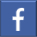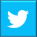# Python Time Module

## This blog gives in-depth knowledge of the various functions available in the Python time module and how these functions can be used. Read our blog to learn more...Jan 26, 2023    By Team YoungWonks *

## Introduction

Python programming language is said to be one of the simplest programming language. This programming language is easy to learn and convenient to use because of the in-built modules. With the help of in-built libraries users are able to create applications and algorithms at a much faster rate compared to other programming languages. It consists of simple libraries such as random, time, datetime, calendar whereas it consists of libraries such as flask, Django, numpy, pandas which helps the user to work on web development and data analytics.

In this blog tutorial we will discuss about the functionality of one such module known as the time module. We will look into detail about the functions and its application.

## What is Python time module?

As the name suggests, Python time module is used to create time related tasks in the programs.

It provides direct functions which can be used to display time in different units and various ways such as strings, numbers. It also has related functions to format the programs based on time such as sleep function.

## How to use the Python time module?

Python time module is an in-built module therefore it comes pre-installed with the Python package. In order to use the time module we need to only import this module.

Syntax:

import time

## Functions of the Python time module

Let us now into the time functions of this module.

### 1. Sleep function

This function helps the program to wait when the code is getting executed. Number of seconds of wait is added as an argument.

Syntax:

time.sleep(n)

Here n is the number of seconds.

In the example given above, the code waits for 2 seconds after printing Hello.

### 2. Time function

This function gives the number of seconds since epoch.

Epoch: This is a reference point when the time starts and is marked as the start time of an era. An era is a fixed point in time which is used to calculate time. Therefore in Python time epoch is the starting point. It uses this point to calculate the current time. This is platform-dependent. The time since the epoch is represented in number of seconds.

The code given below is used to check the epoch on a given platform. Usually, epoch on most of the platforms such as Windows, UNIX systems is January 1, 1970, 00:00:00 Coordinated Universal Time (UTC). Leap seconds are not counted since the epoch. It is a one-second adjustment to made to the UTC time. UTC is also known as Greenwich Mean time (GMT).

Syntax:

time.gmtime(0)

If you notice the output obtained in the above image there are a few values such as dst, mday assigned to a few arguments. Let us discuss about a few of them.

mon = Month number

mday = day of the month

wday = week day represented as an integer (Monday is 0 as it is considered as the first day of the week and so on)

yday = day number based on year

isdst = returns True or False for Daylight Savings Time

### 3. Time Function

The code given below is used to derive the current time since epoch. This function returns the time in number of seconds. Number of seconds is returned as a floating point number.

Syntax:

time.time()

The time before epoch can also be represented with a negative sign.

### 4. Local time Function

This function returns struct_time object which contains a tuple having few attributes. The struct_time class is the same which we have obtained in one of the above functions. It takes the number of seconds since epoch as the argument. Take a look at the example given below.

Syntax:

time.localtime(n)

Look at the other two print statements which makes use of the attribute names to get the values of specific attributes.

### 5. Current time function

The return value of this function is the current year, date and time along with the day. This also takes the number of seconds since epoch as the argument.

Syntax:

time.ctime (n)

Until now we have seen how we get the struct_time object. Now let us learn how to do the other way around. We will make use of a time tuple which is a named tuple.

Note: If no argument is present it calculates the time till present.

### 6. Function to convert time string

This function takes a time string as an argument and returns a struct_time.

Syntax:

string = ‘given time‘

time.strptime (string)

### 7. Functions to convert struct_time object

The below function parses a time tuple to obtain the number of seconds since epoch and current time. Here time tuple is named on the basis of the attributes present in it.

Number of Seconds:

Syntax:

tuple = (year, month, day, hour, minute, second, weekday, day of the year, daylight saving)

time.mktime (tuple)

Note: The structure of the tuple should be followed i.e. the order of the attributes.

Current time:

Syntax:

tuple = (year, month, day, hour, minute, second, weekday, day of the year, daylight saving)

time.asctime (tuple)

The above function returns the current date and time. The output obtained is a time string and is a human-readable string.

### 8. String format time function

This function takes 2 parameter. The struct_time object is one parameter and returns a time string. The string can have several information such as the date, time, year in any order. The order is decided based on the format code given as an parameter to the function. Format code is the time format desired by the user.

Syntax:

t = time.localtime(n)

time.strftime(‘string format‘, t)

*Contributors: Written by Aayushi Jayaswal; Lead image by Shivendra Singh

This blog is presented to you by YoungWonks. The leading coding program for kids and teens.

YoungWonks offers instructor led one-on-one online classes and in-person classes with 4:1 student teacher ratio.

Sign up for a free trial class by filling out the form below:

By clicking the "Submit" button above, you agree to the privacy policyShare on FacebookShare on Twitter
help